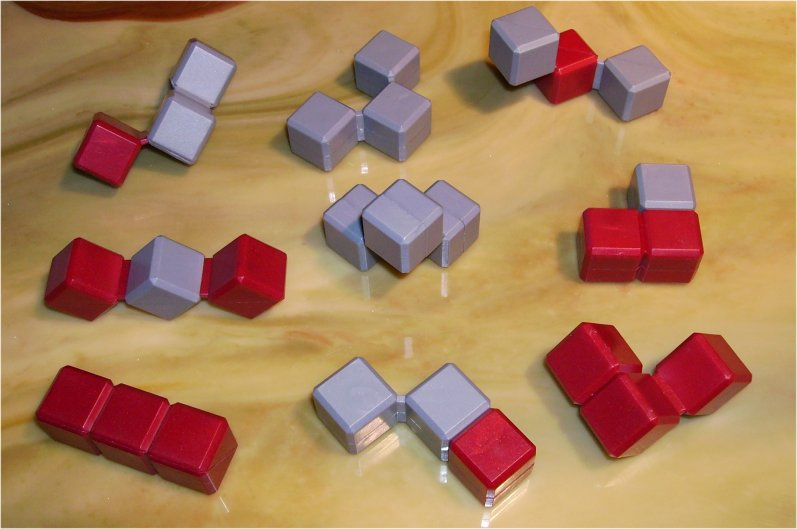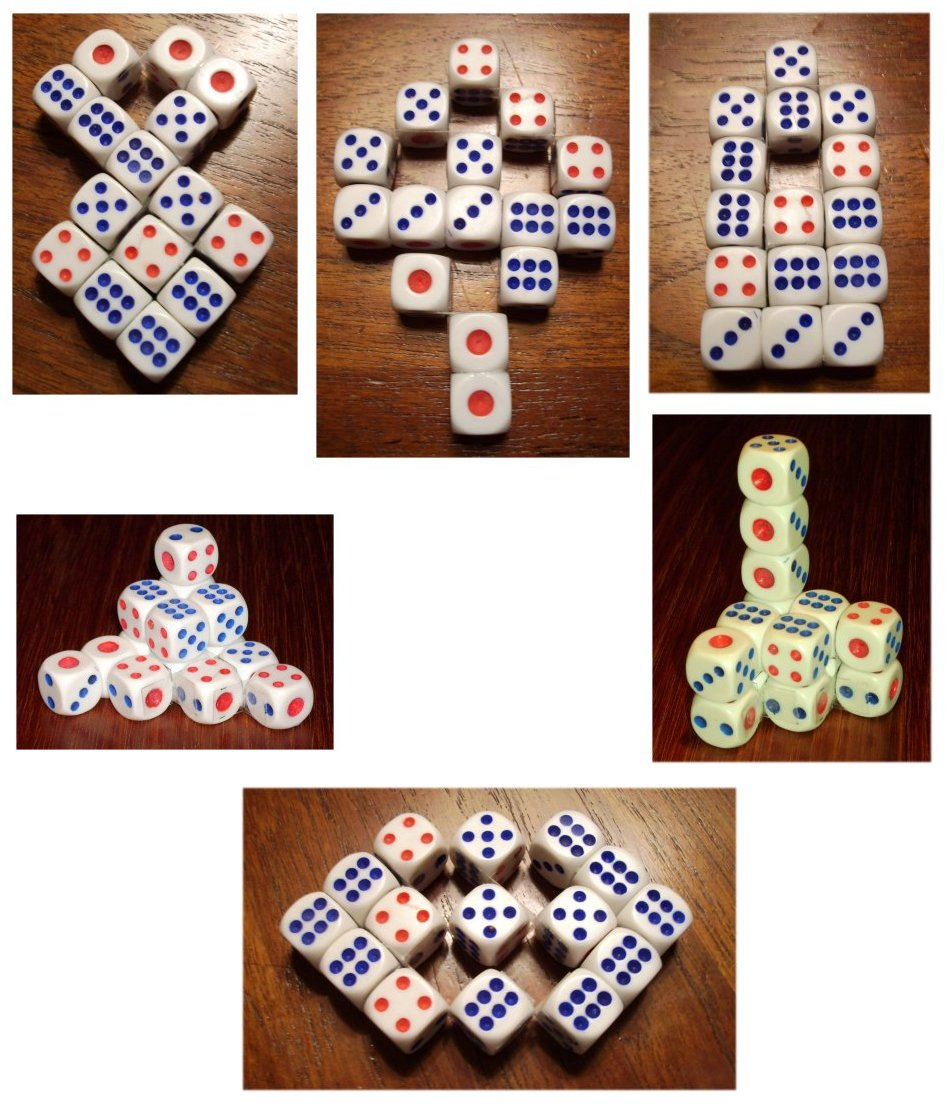# Pseudo-Tricubes:

### 3-dim Pseudo-Tricubes:

There are 9 pseudo-tricubes with a total volume of 27 cubes.A 3x3x3 cube can be made form these pieces. This is a nice puzzle which is available in trade. Three other possible constructions are shown.Solutions for these figures are
here.

### 2-dim Pseudo-Tricubes:

If you only use the five flat pieces with a total of 15 cubes you may ask for a 3x5 rectangle but this isn't possible. Instead of you can try for some symmetric figures in two or three dimensionsIndex
Home